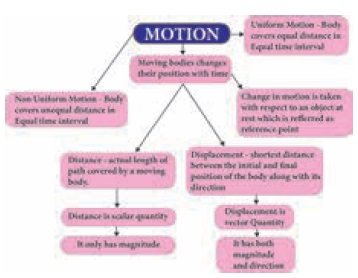Home | | Science 9th std | Distance and Displacement

# Distance and Displacement

The actual length of the path travelled by a moving body irrespective of the direction is called the distance travelled by the body.

Distance and Displacement

## 1. Distance

The actual length of the path travelled by a moving body irrespective of the direction is called the distance travelled by the body. It is measured in metre in SI system. It is a scalar quantity having magnitude only.

## 2. Displacement

It is de ned as the change in position of a moving body in a particular direction. It is vector quantity having both magnitude and direction. It is also measured in metre in SI system.Tags : Science , 9th Science : Motion
Study Material, Lecturing Notes, Assignment, Reference, Wiki description explanation, brief detail
9th Science : Motion : Distance and Displacement | Science